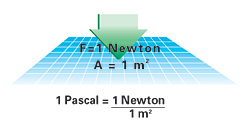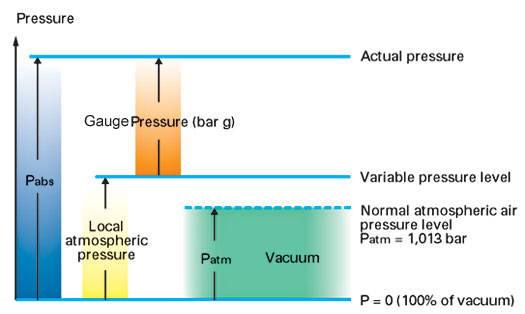# Aluminum for Compressed Air Network## Aluminum for CompressedAir Network

#### Air guide:

Pressure
Pressure is the balance of power on a defined surface area. Pascal (Pa) represents the pressure unit of the International System of Units (SI), but in industrial practice, pressure is represented in pounds per square inch (PSI).

1 bar = 105 Pa =14.5 PSI

1 Pascal=1 Newton
1 m2

1 PSI=1 pound
1 in 2Normal atmospheric air pressure is stated as 14.7 PSI at sea level. Generally used as a reference for pressure measurement, it is, however variable according to altitude. For tests and measurements, it is advisable
to use absolute PSI or bar corresponding to absolute pressure.

Pabs = Patm + Prel
Pabs : absolute pressure
Prel : relative gauge pressure
Patm : normal atmospheric pressureTemperature:
The International System of Units (SI) unit of temperature is Kelvin (K). The following formula establishes the connection between Kelvin, degree Celsius (°C), and degree Fahrenheit (°F),the units more commonly used:

T= t + 273.15
t=(tf-32)X5/9

T : absolute temperature(K)
t : temperature (°C)
tf: temperature (°F)

Relative Humidity:
The percentage of relative humidity is the relation between :
- the quantity of water vapour present in a volume of air
- the quantity of water corresponding to the saturation of this same   volume of air (saturation causing condensation of excess water vapour).

The maximum quantity of water which can be absorbed in a volume of air increases with temperature.About Us | Site Map | Privacy Policy | Contact Us| ©2003 FPDS Company - Italian Branch